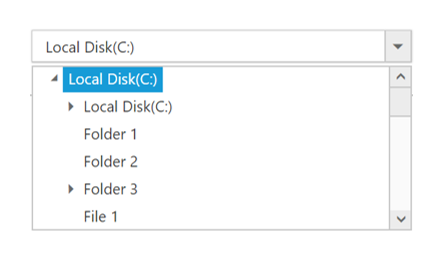Load on demand feature allows the DropDownTree items to load on request from the service/database, on clicking the expand icon. This functionality helps to improve performance on control’s initial rendering time as data items load only on request.

The loadOnDemand property is used to enable or disable the load on demand functionality of the DropDownTree.

• html
• ``<input type="text" id="itemList" />``
• javascript
• ``````// DataManager creation
var dataManager = ej.DataManager({
url: "http://js.syncfusion.com/demos/ejServices/api/TreeViewData/GetAllData",
crossDomain: true,

});

\$(function() {
\$('#itemList').ejDropDownTree({
width: "100%",
treeViewSettings: {
fields: {
dataSource: dataManager,
id: "id",
text: "name",
parentId: "pid",
}
}
});
});``````

You can also bind data from WebAPI controller as shown below and map the WebAPI path to Url field of dataManager.

• c#
• ``````public IEnumerable<Data> Get()
{
List<Data> Items = new List<Data>();
Items.Add(new Data { id = 1, pid = 0, expanded = false, hasChild = true, name = "Local Disk (C:)" });
Items.Add(new Data { id = 2, pid = 1, expanded = false, hasChild = false, name = "Folder 1" });
Items.Add(new Data { id = 3, pid = 1, expanded = false, hasChild = false, name = "Folder 2" });
Items.Add(new Data { id = 4, pid = 1, expanded = false, hasChild = true, name = "Folder 3" });
Items.Add(new Data { id = 20, pid = 4, expanded = false, hasChild = false, name = "File 1" });
Items.Add(new Data { id = 21, pid = 4, expanded = false, hasChild = false, name = "File 2" });
Items.Add(new Data { id = 22, pid = 4, expanded = false, hasChild = false, name = "File 3" });
Items.Add(new Data { id = 5, pid = 0, expanded = false, hasChild = true, name = "Local Disk(D:)" });
Items.Add(new Data { id = 6, pid = 5, expanded = false, hasChild = true, name = "Folder 4" });
Items.Add(new Data { id = 7, pid = 6, expanded = false, hasChild = false, name = "File 4" });
Items.Add(new Data { id = 8, pid = 6, expanded = false, hasChild = false, name = "File 5" });
Items.Add(new Data { id = 9, pid = 6, expanded = false, hasChild = false, name = "File 6" });
Items.Add(new Data { id = 10, pid = 5, expanded = false, hasChild = false, name = "Folder 5" });
Items.Add(new Data { id = 11, pid = 5, expanded = false, hasChild = false, name = "Folder 6" });
Items.Add(new Data { id = 12, pid = 0, expanded = false, hasChild = true, name = "Local Disk(E:)" });
Items.Add(new Data { id = 13, pid = 12, expanded = false, hasChild = false, name = "Folder 7" });
Items.Add(new Data { id = 14, pid = 13, expanded = false, hasChild = false, name = "File 7" });
Items.Add(new Data { id = 15, pid = 13, expanded = false, hasChild = true, name = "File 8" });
Items.Add(new Data { id = 16, pid = 13, expanded = false, hasChild = false, name = "File 9" });
Items.Add(new Data { id = 17, pid = 12, expanded = false, hasChild = false, name = "Folder 8" });
Items.Add(new Data { id = 18, pid = 12, expanded = false, hasChild = true, name = "Folder 9" });
return Items;
}

public class Data
{
public int id;

public int pid;

public bool expanded;

public bool hasChild;

public string name;
}``````